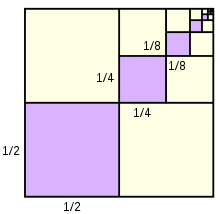# The sum 21

The sum of a geometric progression's 2nd and 3rd terms is six times the 4th term. Find the two possible values of the common ratio B. If the second term is eight, the common ratio is positive. Find the first six terms.

q1 =  0.6404
q2 =  -0.3904
g1 =  12.4922
g2 =  8
g3 =  5.1232
g4 =  3.2809
g5 =  2.1011
g6 =  1.3455

### Step-by-step explanation:Did you find an error or inaccuracy? Feel free to write us. Thank you!

Tips for related online calculators
Are you looking for help with calculating roots of a quadratic equation?